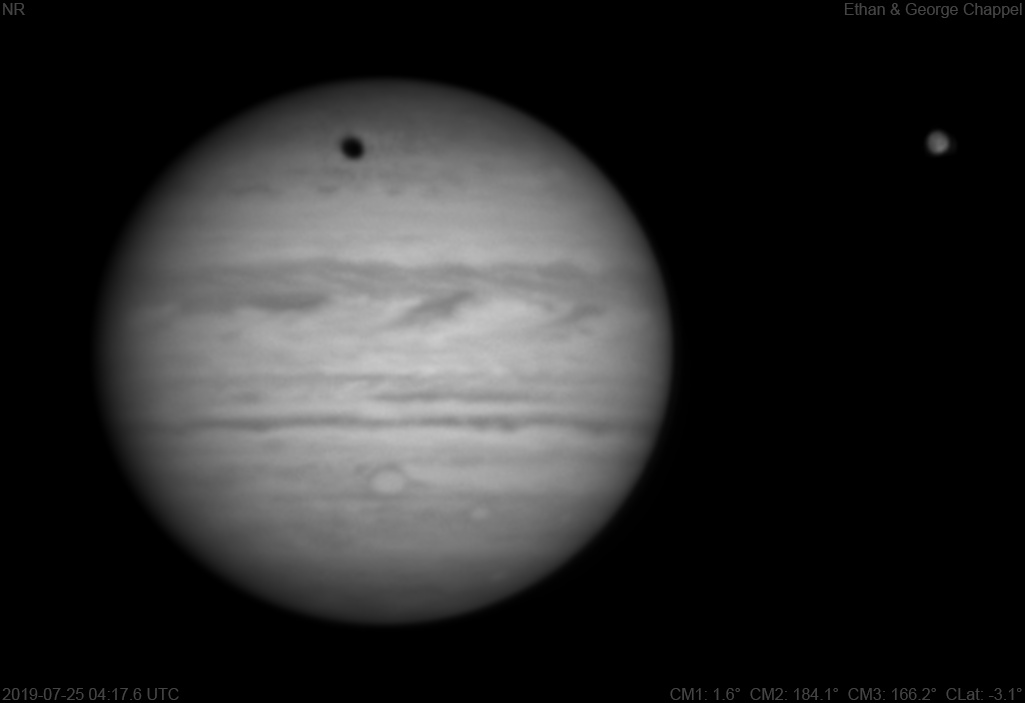# Jupiter 2019-07-25 04:17 UTC

CM1: 1.60°

CM2: 184.10°

CM3: 166.20°

CLat: -3.10°

Description

Less windy conditions allowed us to use the CGEM DX/C14 for the first time in nine days. Seeing was worse than most other nights this summer.

Notes:

• Oval BA is on the central meridian.
• The South Polar Red Spot is about to set.
• Ganymede is showing a few surface details despite the low resolution. Osiris is visible as a bright region in the southern hemisphere.
• Ganymede's shadow is crossing the northern hemisphere.

Equipment

ZWO ASI290MM

Celestron EdgeHD 14

Logs
```FireCapture v2.6  Settings
------------------------------------
Observer=Ethan Chappel
Camera=ZWO ASI290MM
Filter=IR
Profile=Jupiter
Diameter=43.38"
Magnitude=-2.46
CMI=3.6° CMII=186.1° CMIII=168.2°  (during mid of capture)
FocalLength=7900mm (F/28)
Resolution=0.08"
Filename=2019-07-25-0420_5-EC-IR-Jup.ser
Date=2019_07_25
Start=04_19_32.166
Mid=04_20_32.172
End=04_21_32.179
Start(UT)=04_19_32.166
Mid(UT)=04_20_32.172
End(UT)=04_21_32.179
Duration=120.013s
Date_format=yyyy_MM_dd
Time_format=HH_mm_ss
LT=UT -6h
Frames captured=7997
File type=SER
Binning=no
Bit depth=8bit
Debayer=no
ROI=788x632
ROI(Offset)=0x0
FPS (avg.)=66
Shutter=15.00ms
Gain=300 (50%)
AutoExposure=off
SoftwareGain=10 (off)
Brightness=1 (off)
FPS=100 (off)
AutoGain=off
AutoHisto=75 (off)
Gamma=50
HighSpeed=off
USBTraffic=40 (off)
Histogramm(min)=0
Histogramm(max)=147
Histogramm=57%
Noise(avg.deviation)=1.48
AutoAlign=false
PreFilter=none
Limit=120 Seconds
Sensor temperature=31.3°C
Focuser position=3684
FireCapture v2.6  Settings
------------------------------------
Observer=Ethan Chappel
Camera=ZWO ASI290MM
Filter=IR
Profile=Jupiter
Diameter=43.38"
Magnitude=-2.46
CMI=1.8° CMII=184.3° CMIII=166.5°  (during mid of capture)
FocalLength=8800mm (F/31)
Resolution=0.07"
Filename=2019-07-25-0417_6-EC-IR-Jup.ser
Date=2019_07_25
Start=04_16_39.277
Mid=04_17_39.279
End=04_18_39.282
Start(UT)=04_16_39.277
Mid(UT)=04_17_39.279
End(UT)=04_18_39.282
Duration=120.005s
Date_format=yyyy_MM_dd
Time_format=HH_mm_ss
LT=UT -6h
Frames captured=7997
File type=SER
Binning=no
Bit depth=8bit
Debayer=no
ROI=1300x636
ROI(Offset)=0x0
FPS (avg.)=66
Shutter=15.00ms
Gain=300 (50%)
AutoExposure=off
SoftwareGain=10 (off)
Brightness=1 (off)
FPS=100 (off)
AutoGain=off
AutoHisto=75 (off)
Gamma=50
HighSpeed=off
USBTraffic=40 (off)
Histogramm(min)=0
Histogramm(max)=146
Histogramm=57%
Noise(avg.deviation)=1.51
AutoAlign=false
PreFilter=none
Limit=120 Seconds
Sensor temperature=31.3°C
Focuser position=3624
```